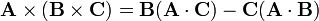# Baccab formula

In vector analysis, the baccab formula gives the expansion of a vector triple product$\mathbf{A}\times(\mathbf{B}\times\mathbf{C}) = \mathbf{B}(\mathbf{A}\cdot\mathbf{C}) -\mathbf{C}(\mathbf{A}\cdot\mathbf{B})$

where the symbol "×" stands for a cross product between two vectors (resulting into a vector) and the symbol "•" stands for an inner product of two vectors (resulting into a scalar).

The name of the formula is because of the mnemonics: "abc = baccab".